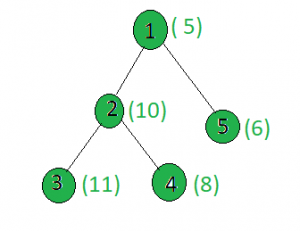# Count the nodes in the given tree whose weight is even

• Last Updated : 22 Apr, 2021

Given a tree, and the weights of all the nodes, the task is to count the number of nodes whose weight is even.
Examples:

Input:Output:
Only the weights of the nodes 2, 4 and 5 are even.

Approach: Perform dfs on the tree and for every node, check if it’s weight is divisible by 2 or not. If yes then increment count.
Below is the implementation of the above approach:

## C++

 `// C++ implementation of the approach``#include ``using` `namespace` `std;` `int` `ans = 0;` `vector<``int``> graph;``vector<``int``> weight(100);` `// Function to perform dfs``void` `dfs(``int` `node, ``int` `parent)``{``    ``// If weight of the current node is even``    ``if` `(weight[node] % 2 == 0)``        ``ans += 1;` `    ``for` `(``int` `to : graph[node]) {``        ``if` `(to == parent)``            ``continue``;``        ``dfs(to, node);``    ``}``}` `// Driver code``int` `main()``{``    ``int` `x = 15;` `    ``// Weights of the node``    ``weight = 5;``    ``weight = 10;``    ``weight = 11;``    ``weight = 8;``    ``weight = 6;` `    ``// Edges of the tree``    ``graph.push_back(2);``    ``graph.push_back(3);``    ``graph.push_back(4);``    ``graph.push_back(5);` `    ``dfs(1, 1);` `    ``cout << ans;` `    ``return` `0;``}`

## Java

 `// Java implementation of the approach``import` `java.util.*;` `class` `GFG``{``    ``static` `int` `ans = ``0``;``    ` `    ``@SuppressWarnings``(``"unchecked"``)``    ``static` `Vector[] graph = ``new` `Vector[``100``];``    ``static` `int``[] weight = ``new` `int``[``100``];``    ` `    ``// Function to perform dfs``    ``static` `void` `dfs(``int` `node, ``int` `parent)``    ``{``        ``// If weight of the current node is even``        ``if` `(weight[node] % ``2` `== ``0``)``            ``ans += ``1``;``    ` `        ``for` `(``int` `to : graph[node])``        ``{``            ``if` `(to == parent)``                ``continue``;``            ``dfs(to, node);``        ``}``    ``}``    ` `    ``// Driver code``    ``public` `static` `void` `main(String[] args)``    ``{``        ``int` `x = ``15``;``    ` `    ``for` `(``int` `i = ``0``; i < ``100``; i++)``            ``graph[i] = ``new` `Vector<>();``        ` `        ``// Weights of the node``        ``weight[``1``] = ``5``;``        ``weight[``2``] = ``10``;``        ``weight[``3``] = ``11``;``        ``weight[``4``] = ``8``;``        ``weight[``5``] = ``6``;``    ` `        ``// Edges of the tree``        ``graph[``1``].add(``2``);``        ``graph[``2``].add(``3``);``        ``graph[``2``].add(``4``);``        ``graph[``1``].add(``5``);``    ` `        ``dfs(``1``, ``1``);``    ` `        ``System.out.println(ans);``    ``}``}` `// This code is contributed by shubhamsingh10`

## Python3

 `# Python3 implementation of the approach``ans ``=` `0` `graph ``=` `[[] ``for` `i ``in` `range``(``100``)]``weight ``=` `[``0``] ``*` `100` `# Function to perform dfs``def` `dfs(node, parent):``    ``global` `ans``    ` `    ``# If weight of the current node is even``    ``if` `(weight[node] ``%` `2` `=``=` `0``):``        ``ans ``+``=` `1``    ` `    ``for` `to ``in` `graph[node]:``        ``if` `(to ``=``=` `parent):``            ``continue``        ``dfs(to, node)` `# Driver code``x ``=` `15` `# Weights of the node``weight[``1``] ``=` `5``weight[``2``] ``=` `10``weight[``3``] ``=` `11``weight[``4``] ``=` `8``weight[``5``] ``=` `6` `# Edges of the tree``graph[``1``].append(``2``)``graph[``2``].append(``3``)``graph[``2``].append(``4``)``graph[``1``].append(``5``)` `dfs(``1``, ``1``)``print``(ans)` `# This code is contributed by SHUBHAMSINGH10`

## C#

 `// C# implementation of the approach``using` `System;``using` `System.Collections.Generic;` `class` `GFG``{``    ``static` `int` `ans = 0;``    ``static` `List<``int``>[] graph = ``new` `List<``int``>;``    ``static` `int``[] weight = ``new` `int``;``     ` `    ``// Function to perform dfs``    ``static` `void` `dfs(``int` `node, ``int` `parent)``    ``{``        ``// If weight of the current node is even``        ``if` `(weight[node] % 2 == 0)``            ``ans += 1;``     ` `        ``foreach` `(``int` `to ``in` `graph[node])``        ``{``            ``if` `(to == parent)``                ``continue``;``            ``dfs(to, node);``        ``}``    ``}``     ` `    ``// Driver code``    ``public` `static` `void` `Main(String[] args)``    ``{     ``        ``for` `(``int` `i = 0; i < 100; i++)``            ``graph[i] = ``new` `List<``int``>();``         ` `        ``// Weights of the node``        ``weight = 5;``        ``weight = 10;``        ``weight = 11;``        ``weight = 8;``        ``weight = 6;``     ` `        ``// Edges of the tree``        ``graph.Add(2);``        ``graph.Add(3);``        ``graph.Add(4);``        ``graph.Add(5);``     ` `        ``dfs(1, 1);``     ` `        ``Console.WriteLine(ans);``    ``}``}` `// This code is contributed by Rajput-Ji`

## Javascript

 ``
Output:
`3`

Complexity Analysis:

• Time Complexity : O(N).
In dfs, every node of the tree is processed once and hence the complexity due to the dfs is O(N) if there are total N nodes in the tree. Therefore, the time complexity is O(N).
• Auxiliary Space : O(1).
Any extra space is not required, so the space complexity is constant.

My Personal Notes arrow_drop_up